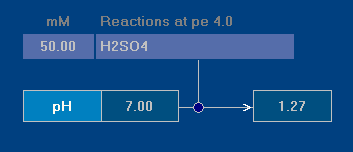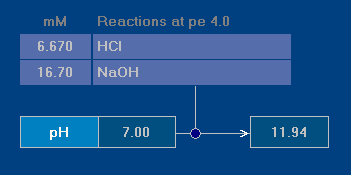# Dilution and Mixing

Problem 1:  Dilution of Sulfuric Acid

40 mL of 2.5 molar H2SO4 solution will be diluted to 2 L. What is the pH of the diluted solution?

Before we enter the parameters into the program, please recap: The dilution factor is

2000 mL / 40 mL  =  50

Hence, the diluted solution contains exactly (2500 mM / 50) = 50 mM H2SO4.Switch to pure water (button H2O), then click on Reac and enter for “H2SO4” the value 50 mM.

After clicking on Start the program outputs the result (see right screenshot):

pH = 1.27

The diluted solution has pH 1.27.

Note. If you would solve this task with a pocket calculator, you would get a pH value of 1.3. The deviation from the correct result (pH 1.27) is caused by activity corrections for non-ideal solutions.

Problem 2:  Mixing HCl with NaOH

100 mL of 0.01 molar HCl is mixed with 50 mL of 0.05 molar NaCl. What is the pH of the final solution?